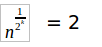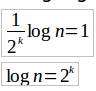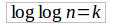Open in App
Not now

# GATE | GATE-CS-2002 | Question 36

• Last Updated : 28 Jun, 2021

The running time of the following algorithm Procedure A(n)

```  If n < = 2 return (1)
else return (A(Image not present√nImage not present));```

is best described by :
(A) O(n)
(B) O(log n)
(C) O(log Log n)
(D) O(l1)

Explanation:

Now let the function is called k timesTaking log on both side (base 2).Taking log again.Running time complexity = number of function calls = k = Therefore Running time complexity =.

See question 5 of https://www.geeksforgeeks.org/data-structures-and-algorithms-set-11/

This solution is contributed by Parul Sharma.

My Personal Notes arrow_drop_up
Related Articles